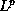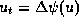## References

1
C. BARDOS, F. GOLSE & D. LEVERMORE, Fluid dynamic limits of kinetic equations II: convergence proofs of the Boltzmann equations, Comm. Pure Appl. Math. XLVI (1993), 667-754.
2
Y. BRENIER, Résolution d'équations d'évolution quasilinéaires en dimension N d'espace à l'aide d'équations linéaires en dimension N+1, J. Diff. Eq. 50 (1983), 375-390.
3
G.-Q. CHEN, Q. DU & E. TADMOR, Spectral viscosity approximation to multidimensional scalar conservation laws, Math. of Comp. 57 (1993).
4
B. COCKBURN, F. COQUEL & P. LEFLOCH, Convergence of finite volume methods for multidimensional conservation laws, SIAM J. Numer. Anal. 32 (1995), 687-705.
5
C. CERCIGNANI, The Boltzmann Equation and its Applications, Appl. Mathematical Sci. 67, Springer, New-York, 1988.
6
G.-Q. CHEN, The theory of compensated compactness and the system of isentropic gas dynamics, Preprint MCS-P154-0590, Univ. of Chicago, 1990.
7
R. DIPERNA, Convergence of the viscosity method for isentropic gas dynamics, Comm. Math. Phys. 91 (1983), 1-30.
8
R. DIPERNA, Measure-valued solutions to conservation laws, Arch. Rat. Mech. Anal. 88 (1985), 223-270.
9
R. DIPERNA & P. L. LIONS, On the Cauchy problem for Boltzmann equations: Global existence and weak stability, Ann. Math. 130 (1989), 321-366.
10
R. DIPERNA & P.L. LIONS, Global weak solutions of Vlasov-Maxwell systems, Comm. Pure Appl. Math. 42 (1989), 729-757.
11
R. DIPERNA, P.L. LIONS & Y. MEYER,regularity of velocity averages, Ann. I.H.P. Anal. Non Lin. 8(3-4) (1991), 271-287.
12
P. G´ERARD, Microlocal defect measures, Comm. PDE 16 (1991), 1761-1794.
13
F. GOLSE, P. L. LIONS, B. PERTHAME & R. SENTIS, Regularity of the moments of the solution of a transport equation, J. of Funct. Anal. 76 (1988), 110-125.
14
Y. GIGA & T. MIYAKAWA, A kinetic construction of global solutions of first-order quasilinear equations, Duke Math. J. 50 (1983), 505-515.
15
C. JOHNSON & A. SZEPESSY, Convergence of a finite element methods for a nonlinear hyperbolic conservation law, Math. of Comp. 49 (1988), 427-444.
16
C. JOHNSON, A. SZEPESSY & P. HANSBO, On the convergence of shock-capturing streamline diffusion finite element methods for hyperbolic conservation laws, Math. of Comp. 54 (1990), 107-129.
17
Y. KOBAYASHI, An operator theoretic method for solving, Hiroshima Math. J. 17 (1987) 79-89.
18
D. KRfONER, S. NOELLE & M. ROKYTA, Convergence of higher order upwind finite volume schemes on unstructured grids for scalar conservation laws in several space dimensions, Numer. Math. 71 (1995) 527-560.
19
D. KRfONER & M. ROKYTA, Convergence of Upwind Finite Volume Schemes for Scalar Conservation Laws in two space dimensions, SINUM 31 (1994) 324-343.
20
P.D. LAX, Hyperbolic Systems of Conservation Laws and the Mathematical Theory of Shock Waves (SIAM, Philadelphia, 1973).
21
P. L. LIONS, B. PERTHAME & P. SOUGANIDIS, Existence and stability of entropy solutions for the hyperbolic systems of isentropic gas dynamics in Eulerian and Lagrangian coordinates, Comm. Pure and Appl. Math. 49 (1996), 599-638.
22
P. L. LIONS, B. PERTHAME & E. TADMOR, Kinetic formulation of scalar conservation laws and related equations, J. Amer. Math. Soc. 7(1) (1994), 169-191
23
P. L. LIONS, B. PERTHAME & E. TADMOR, Kinetic formulation of the isentropic gas-dynamics equations and p-systems, Comm. Math. Phys. 163(2) (1994), 415-431.
24
S. NOELLE & M. WESTDICKENBERG Convergence of finite volume schemes. A new convergence proof for finite volume schemes using the kinetic formulation of conservation laws, Preprint.
25
O. A. OL\V EINIK Discontinuous solutions of nonlinear differential equations, Amer. Math. Soc. Transl. (2), 26 (1963), 95-172.
26
B. PERTHAME, Global existence of solutions to the BGK model of Boltzmann equations, J. Diff. Eq. 81 (1989), 191-205.
27
B. PERTHAME, Second-order Boltzmann schemes for compressible Euler equations, SIAM J. Num. Anal. 29, (1992), 1-29.
28
B. PERTHAME & E. TADMOR, A kinetic equation with kinetic entropy functions for scalar conservation laws, Comm. Math. Phys.136 (1991), 501-517.
29
K. H. PRENDERGAST & K. XU, Numerical hydrodynamics from gas-kinetic theory, J. Comput. Phys. 109(1) (1993), 53-66.
30
J. SMOLLER, Shock Waves and Reaction-Diffusion Equations, Springer-Verlag, New York, 1983.
31
L. TARTAR, Compensated compactness and applications to partial differential equations, in Research Notes in Mathematics 39, Nonlinear Analysis and Mechanics, Heriott-Watt Symposium, Vol. 4 (R.J. Knopps, ed.) Pittman Press, (1975), 136-211.
32
L. TARTAR, Discontinuities and oscillations, in Directions in PDEs, Math Res. Ctr Symposium (M.G. Crandall, P.H. Rabinowitz and R.E. Turner eds.) Academic Press (1987), 211-233.